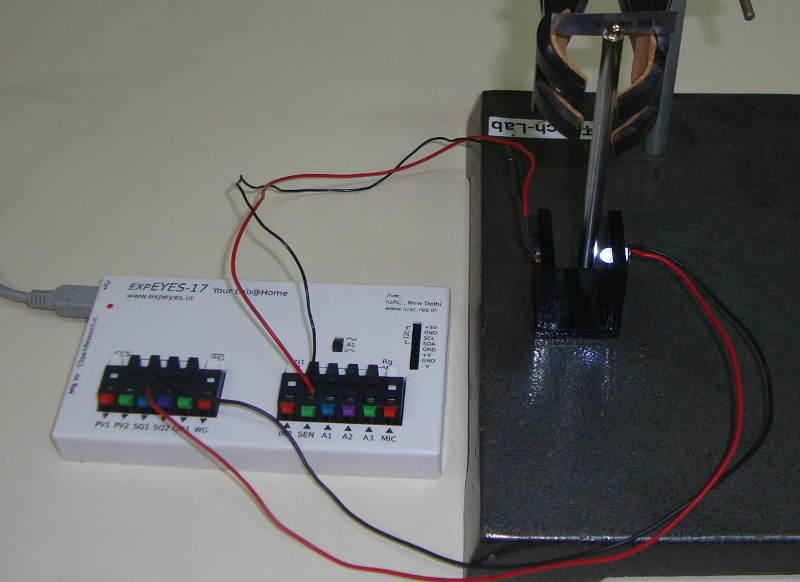# Measuring Acceleration due to Gravity

## Using a pendulum to calculate the value of gBrief Summary
A rod pendulum is allowed to freely oscillate between a phototransistor and an LED, and ExpEYES accurately measures its period of oscillations. Its length is measured, and the value of acceleration due to gravity applicable for the measured time period is calculated.

## Experiment detailsSQ1 is set to HIGH (5V), and an LED is connected to it. Since SQ1 has a current limiter inside, the LED will not burn out.

On the other side, a phototransistor is connected between SEN and GROUND. When light is incident on the phototransistor, it conducts well, and the value of SEN drops to near zero volts (LOW).

When the pendulum interrupts the LED’s light from falling on the phototransistor, the signal rises to 3.3V (HIGH)

In one oscillation, the pendulum interrupts the light twice, cause two corresponding rising edge signals on SEN. This is measured and treated as the time for a single oscillation.Using the well known formula for a rod pendulum:

$T = 2*pi*\frac{2*m*L^2}{3*m*g*L} = 2*pi*\frac{2*L}{3*g}$

We can measure L using a scale, T using expeyes, and calculate the third variable g .

### Rod pendulum with a blade to create a frictionless pivot